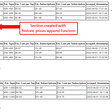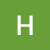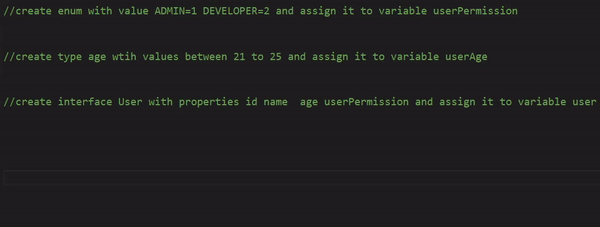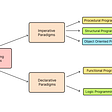# Solving Popular Algorithms: Matrix Diagonal Sums

`mat = [[1,2,3],[4,5,6],[7,8,9]]`
`diagonalSum = (mat) => {   let primSum = 0      for (let i = 0; i < mat.length; i++) {      primSum += mat[i][i]   } }`
`diagonalSum = (mat) => {   let primSum = 0   let secSum = 0   for (let i = 0; i < mat.length; i++) {      primSum += mat[i][i]      secSum += mat[i][mat.length-1-i]   } }`
`diagonalSum = (mat) => {   let primSum = 0   let secSum = 0   for (let i = 0; i < mat.length; i++) {      primSum += mat[i][i]      secSum += mat[i][mat.length-1-i]   }      if (mat.length%2 !== 0) {      let mid = Math.floor(mat.length/2)      secSum -= mat[mid][mid]   }      return primSum + secSum}`

--

--

--

## More from HopeGiometti

Love podcasts or audiobooks? Learn on the go with our new app.

## Telerik Platform retires. Here are your alternatives## Possible Solutions For Requirements Creep## Ulord Project Progress (From October 29 to November 4, 2020)## How we empower developers with the right tools, platforms and services for a modern Cloud journey## How to keep up to date as a C# developer## How to Create a Python Script to Automatically Monitor Prices Online## C Programming Hacks 2: Efficiently Reading a File Line-by-line## GitHub Copilot — AI pair programmer## Programming 101: Declarative vs Imperative Paradigm## How does recursion work?## Code refactoring in Delphi